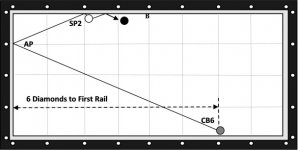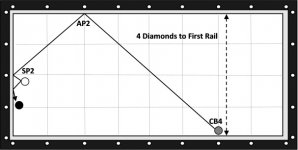# For Dr. Larry "A Kicking System"

#### mr3cushion

##### Verified Member
I know He's a 'system nut' so here's one for you Larry that I developed many years ago.

It has some different Math involved, but you'll get it.

BTW, this is a excerpt form one of a few a 3C clinics I held at, Southside Billiards in Savannah, GA. between 2012-13.

In Diagram 2, the calculation is as follows, (CB6) + (SP2) = 8, (SP2)/ (CB6) + (SP2) = 2/8 the fraction reduced = ¼, divide the distance (CB6) lies across the table from (SP2) which is 4 diamonds, ¼ of 4 diamonds = 1 (AP1) aiming point on 1st Cushion.

Note: The English applied in this system is consistently 12 O’clock English. A Moderate-Soft, Normal Stroke is used.

BTW, How to tell when a numeric system is Really a 'Mathematical' system. When the system actually works mathematically, 'Across & the Length' of the table in the correct Aspect Ratio.The next example in Diagram 3 shows the system hitting the long rail first. The calculation is simple, cue ball position (CB4) + scoring point (SP2) = 6, (SP2) / (CB4) + (SP2) = 2/6, reduced = 1/3, divide the distance (CB4) lies from the end of table from (SP2), which is 6 diamonds, 1/3 of 6 diamonds = 2 (AP2), aiming point on 1st Cushion.Last edited:
•lll

#### hankh

##### Verified Member
Mr 3c. Howdy;

Interesting thoughts there. Will need to look into it some more later.
Thanks for the information.

hank

#### lll

##### Verified Member
thanks for sharing bill …#### 12squared

##### Verified Member
I know He's a 'system nut' so here's one for you Larry that I developed many years ago.

It has some different Math involved, but you'll get it.

BTW, this is a excerpt form one of a few a 3C clinics I held at, Southside Billiards in Savannah, GA. between 2012-13.

In Diagram 2, the calculation is as follows, (CB6) + (SP2) = 8, (SP2)/ (CB6+SP2) = 2/8 the fraction reduced = ¼, divide the distance (CB6) lies across the table from (SP2) which is 4 diamonds, ¼ of 4 diamonds = 1 (AP1) aiming point on 1st Cushion.

Note: The English applied in this system is consistently 12 O’clock English. A Moderate-Soft, Normal Stroke is used.

BTW, How to tell when a numeric system is Really a 'Mathematical' system. When the system actually works mathematically, 'Across & the Length' of the table in the correct Aspect Ratio.

View attachment 437986

The next example in Diagram 3 shows the system hitting the long rail first. The calculation is simple, cue ball position (CB4) + scoring point (SP2) = 6, (SP2) / (CB4+SP2) = 2/6, reduced = 1/3, divide the distance (CB4) lies from the end of table from (SP2), which is 6 diamonds, 1/3 of 6 diamonds = 2 (AP2), aiming point on 1st Cushion.

View attachment 437987

Interesting Bill. I fixed your equations in red as you must add the two points before you divide. I hope I'm right hahahaha. I think it reads better now?

Bring some throat lozenges next time or drink some waterLast edited:

#### mr3cushion

##### Verified Member
Interesting Bill. I fixed your equations in red as you must add the two points before you divide. I hope I'm right hahahaha. I think it reads better now?

Bring some throat lozenges next time or drink some waterDave, this is the 'symbol ("/") for "out of' or the word, "over" as in creating a fraction.

#### 12squared

##### Verified Member
Dave, this is the 'symbol ("/") for "out of' or the word, "over" as in creating a fraction.
I understand, but the divide symbol (and the times symbol) get acted on first in an equation like this. So in your example "(SP2)/ (CB6) + (SP2) = 2/8" it would read 2/6 = 1/3 + 2 = 2 1/3 instead of 1/4 like you intended. If it read like 2/(6+2) that would be 2/8 as the the division would be the sum of the 6 and 2.

No matter, it's a pretty cool system and it was understood once seeing the video. Thanks.

Last edited:

#### hankh

##### Verified Member
mr3c, Howdy;

Thinkin' that 12 squared is referring to the ' Order of Operations';

hank

•lll

#### mr3cushion

##### Verified Member
Maybe I should have posted the simple explanation of the formula.

## The Two-Cushion-First Aiming System​

The calculations for this system are fairly simple. You locate the cue ball position (CB) from the first rail and the point you need to contact the third rail to score the point (SP) from first rail. Then you add the cue ball position (CB) + (SP) = X. You then make a fraction of the scoring point (SP) as the top number of the fraction and the total of the cue ball position (CB) plus the scoring point (SP) which is X, your fraction is SP/CB+SP = X, then divide that into the distance your cue ball (CB) lies across the table from the scoring point (SP) which yields the aiming point (AP) on the 1st Cushion. The aiming point is ALWAYS OPPOSITE the diamond.

Note: The player needs to just remember the principles that apply to each individual system and NOT incorporate other factors or extensions of the systems. The systems work correctly for a reason. Trying to parallel ball lines, applying side spin, using excessive speeds will just alter the results and be ineffective.

Last edited:
•lll